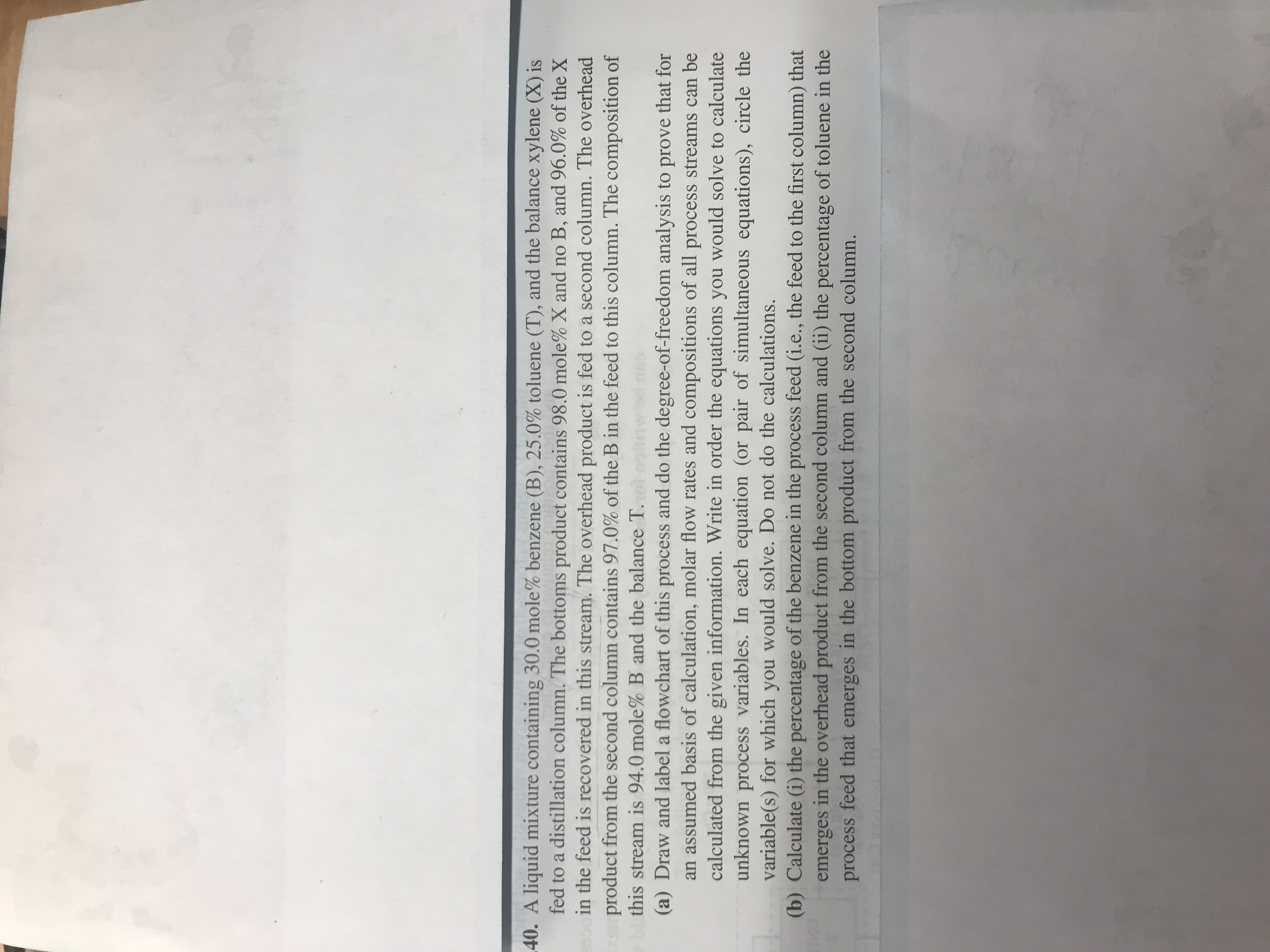# 40. A liquid mixture containing 30.0 mole% benzene (B), 25.0% toluene (T), and the balance xylene (X) is fed to a distillation column. The bottoms product contains 98.0 mole% X and no B, and 96.0% of the X in the feed is recovered in this stream. The overhead product is fed to a second column. The overhead product from the second column contains 97.0% of the B in the feed to this column. The composition of this stream is 94.0 mole% B and the balance T. (a) Draw and label a flowchart of this process and do the degree-of-freedom analysis to prove that for an assumed basis of calculation, molar flow rates and compositions of all process streams can be calculated from the given information. Write in order the equations you would solve to calculate unknown process variables. In each equation (or pair of simultaneous equations), circle the variable(s) for which you would solve. Do not do the calculations. (b) Calculate (i) the percentage of the benzene in the process feed (i.e., the feed to the first column) that emerges in the overhead product from the second column and (ii) the percentage of toluene in the process feed that emerges in the bottom product from the second column.

Questionhelp_outlineImage Transcriptionclose40. A liquid mixture containing 30.0 mole% benzene (B), 25.0% toluene (T), and the balance xylene (X) is fed to a distillation column. The bottoms product contains 98.0 mole% X and no B, and 96.0% of the X in the feed is recovered in this stream. The overhead product is fed to a second column. The overhead product from the second column contains 97.0% of the B in the feed to this column. The composition of this stream is 94.0 mole% B and the balance T. (a) Draw and label a flowchart of this process and do the degree-of-freedom analysis to prove that for an assumed basis of calculation, molar flow rates and compositions of all process streams can be calculated from the given information. Write in order the equations you would solve to calculate unknown process variables. In each equation (or pair of simultaneous equations), circle the variable(s) for which you would solve. Do not do the calculations. (b) Calculate (i) the percentage of the benzene in the process feed (i.e., the feed to the first column) that emerges in the overhead product from the second column and (ii) the percentage of toluene in the process feed that emerges in the bottom product from the second column. fullscreen

### Want to see this answer and more?

Experts are waiting 24/7 to provide step-by-step solutions in as fast as 30 minutes!*